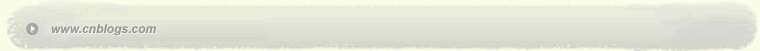Impossible is nothing 爱过知情重醉过知酒浓 　　花开花谢终是空 　　缘份不停留像春风来又走 　　女人如花花似梦

 < 2023年5月 >
30123456
78910111213
14151617181920
21222324252627
28293031123
45678910

• 随笔 - 8
• 文章 - 91
• 评论 - 16
• 引用 - 0

# 相册

•

### 评论排行榜

C++ 的另一个新世界

C++ 的 MetaProgramming

struct hello_world; //forward declaration
struct A : hello_world
{
};

base class hello_world undefined

base class `hello_world' has incomplete type

C++模板的最经常的认识就是STL中的容器, 例如
vector<int> 就是一个可以装int的动态数组, vector<sharp*> 就是一个可以装sharp对象指针的数组.

template<class T> struct Foo {};

template<> struct Foo<int>  {};

template<class T> struct Foo<T*> {}

template<class T, class U> struct bar {};

template<class T> struct bar<T, int>  {};

hello,world的例子以后, 我们准备干点有意思的事情. 例如求两数之和. 首先看看在运行时的函数我们应该如何实现:

int sum(int x, int y) { return x+y; }

int j =  sum(3, 4)

meta programming 由于处于编译器, 因此给它的参数必须是编译时就可以确定的, 在当前C++中允许的为,integer 和 type.

int j = sum_<3,4>::value;

3,4 都为整形常量, 编译时确定, 然后返回值如何取得? 记住在编译时是无法进行真正的函数调用的,因此我们只有通过定义在模板类中的一个常量

sum的定义如下:
template<int x, int y>
struct sum_
{
enum { value = x + y };
};

#include <iostream>

using namespace std;

template<int x, int y>
struct sum_

{

enum { value = x + y };

};

int main()

{

cout << sum_<3, 4>::value << endl;

return 0;

}

int foo(int a, int b)

foo<a, b>::value

sum_<3,4,5>::value

sum( 3, sum(4, 5))

sum_<   //开始参数
sum_< 3 //第一个参数为3
sum_< 3, sum_<   //第二个参数是另外一个表达式的结果
sum_< 3, sum_<4, //这个表达式的第一个参数为４
sum_< 3, sum_<4, 5> //这个表达式的第二个参数为５
sum_< 3, sum_<4, 5>::value //通过::value获得这个表达式的结果
sum_< 3, sum_<4, 5>::value >::value //然后获得整个表达式的结果

ok, 就这么多, 看出来没有, 再解释一次, 将上面我们的
cout << sum_<3, 4>::value << endl;

cout << sum_<3, ?? >::value << endl;

===>
?? =  sum_<4, 5>::value

===> then
cout << sum_<3, sum_<4, 5>::value >::value << endl;

out << sum_< ?? , sum_<4, 5>::value >::value << endl;

?? =  sum_< 2, 3 >::value

cout << sum_< sum_<2, 3>::value , sum_<4, 5>::value >::value << endl;

cout << fac<5>::value ;  // 结果应该是 5 * 4 * 3 * 2 * 1 = 120;

template<int i>
struct fac
{
enum { value = ??? };
};

int fac(int n)
{
if( n == 1)
return 1;
return n * fac(n-1);
}

n *       //n*
fac       //调用下一个fac
fac<n-1>  //参数为n-1
fac<n-1>::value //获得结果

template<int i>
struct fac
{
enum { value = i * fac<i - 1>::value };
};

template<>
struct fac<1>      //参数为1时的特化
{
enum { value = 1 };
};

cout << fac<5>::value << endl;

template<>
struct fac<5>
{
enum { value = 5 * fac<4>::value };
};

easy, 对不?

G++报错     : template instantiation depth exceeds maximum of 500

fac<-1>::value == -1  是成立的.

int safe_fac(int n)
{
if( n < 1)
return -1;
return fac(n);
}

template<int n>
struct safe_fac
{
enum { value =  (n < 1 ? -1 : fac<n>::value ) };
};

template< bool b , class T, class U>
struct if_
{
typedef T type;
};

if_<true, int, double>::type  的结果就是 int 类型, 注意是"类型", 不是对象.

if_<false, int, double>::type 的返回结果是double

template<class T, class U>
struct if_<false, T, U>
{
typedef U type;
};

VC6是支持全部特化的, 因此我们可以将true, false特化出来

template<bool>
struct if_help
{
...
};

template<>
struct if_help<false>
{
...
};

template<bool>
struct if_help
{
template<class T, class U>
struct In
{
typedef T type;
};
};

template<>
struct if_help<false>
{
template<class T, class U>
struct In
{
typedef U type;
};
};

template<bool b, class T, class U>
struct if_
{
typedef if_help<b>::In<T, U>::type type;
};

if_<true, int, double>::type  获得返回的类型了.

typedef if_help<b>::In<T, U>::type type;
===>
typedef typename if_help<b>::template In<T, U>::type type;

if_                          //函数名
if_<true,                    //第一个参数, bool 型
if_<true, int                //第二个参数, 类型
if_<true, int, double        //第3个参数,  类型
if_<true, int, doubble>      //右括号表示参数结束
if_<true, int, double>::type //通过::type获得返回结果, 不是value了, 当然这仅仅是一个命名惯例.

for( if_<true, int, double>::type i = 0; i < 10; i++) {
cout << "line " << i << "\n";
}

for( int i = 0; i < 10; i++) {
cout << "line " << i << "\n";
}

template<int n>
struct safe_fac
{
enum { value = fac<n>::value };
};

enum
{
value = if_< n < 1,???,  fac<n> >::type::value
};

fac<n>类型, 然后通过::type获得返回的类型,或者为???, 或者为fac<n>,

struct return_neg1
{
enum { value = -1 };
};

template<int n>
struct int_
{
enum { value = n };
};

value = if_< n < 1, int_<-1>,  fac<n> >::type::value

template<int n>
struct safe_fac
{
enum { value = if_< n < 1, int_<-1>,  fac<n> >::type::value };
};

cout << safe_fac<-1>::value 输出 -1.

--------------------------------------------------------------------------------
Boost中的mpl (meta programming library) 提供了一个专门用于metaprogramming的library, 同时前面提到的if_, int_等等就

Modern C++ Design 其中的Typelist将meta progamming的循环(就是递归)发挥得淋漓尽致, 在侯捷的网站上www.jjhou.com有免费的前4章可读. Typelist在第三章.

GoF之一的 John Vlissides同样提到了typelists这一章就值得本书的价格. I believe.

posted on 2006-02-27 23:37 笑笑生 阅读(386) 评论(0)  编辑 收藏 引用 所属分类: C++语言Copyright © 笑笑生 Powered by: 博客园 模板提供：沪江博客